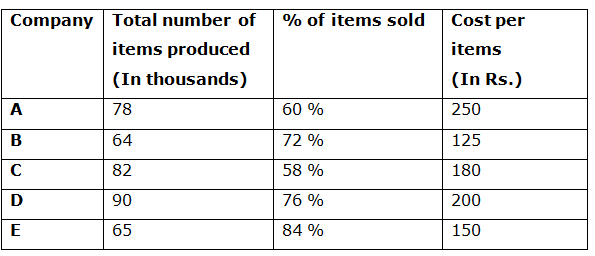# CWC/FCI Prelims 2019 – Quantitative Aptitude Questions (Day-32)

Dear Readers, Exam Race for the Year 2019 has already started, To enrich your preparation here we are providing new series of Practice Questions on Quantitative Aptitude – Section for CWC/FCI Exam. Aspirants, practice these questions on a regular basis to improve your score in aptitude section. Start your effective preparation from the right beginning to get success in upcoming CWC/FCI Exam.

[WpProQuiz 5790]

Directions (1 – 5): What value should come in the place of question mark (?) in the following questions?

1) (4 × 4)3 ÷ (512 ÷ 8)4 × (32 × 8)4 = (4)? + 4

a) 8

b) 5

c) 7

d) 6

e) None of these

2) 76 % of 1285 – 35 % of 1256 =?

a) 537

b) 629

c) 451

d) 787

e) None of these

3) (2/7) of 5033 + 78 % of 650 = (?)2 + 181

a) 48

b) 46

c) 42

d) 39

e) None of these

4) 32 % of 480 + (5/7) of 1890 – 28 % of 820 =?

a) 1336

b) 1178

c) 1452

d) 1274

e) None of these

5) ? *100/(140 × 8 – 680) = 330 + 452

a) 9857

b) 10362

c) 11238

d) 8564

e) None of these

Directions (6 – 10): Study the following information carefully and answer the given questions:

Following table shows the total number items produced by 5 different companies (In thousands) and the percentage of items sold and the cost per items.6) Total number of items sold by the company A is approximately what percentage of total number of items sold by the company C?

a) 100 %

b) 90 %

c) 80 %

d) 115 %

e) 65 %

7) Find the total cost earned by the company B and D together?

a) 1.728 Crore

b) 1.856 Crore

c) 1.642 Crore

d) 1.944 Crore

e) None of these

8) Find the ratio between the total number of items sold by the company B to that of company D?

a) 25: 36

b) 64: 95

c) 52: 77

d) 48: 69

e) None of these

9) Find the difference between the total cost earned by the company A to that of company E?

a) 0.351 Crore

b) 3.51 Crore

c) 0.0351 Crore

d) 35.1 lakhs

e) None of these

10) Total number of items produced by the company A and D together is approximately what percentage more/less than the total number of items produced by the company B and C together?

a) 25 % more

b) 20 % less

c) 15 % more

d) 15 % less

e) 20 % more

Direction (1-5) :

46 ÷ (512/8)4 × (32 × 8)4 = (4)x + 4

46 ÷ 644 × 2564 = (4)x + 4

46 ÷ 412 × 416 = 4x + 4

46 – 12 + 16 = 4x + 4

410 = 4x + 4

x + 4 = 10

x = 6

(76/100)*1285 – (35/100)*1256 = X

X = 976.6 – 439.6

X = 537

(2/7)*5033 + (78/100)*650 = x2 + 181

1438 + 507 – 181 = x2

X2 = 1764

X = 42

(32/100)*480 + (5/7)*1890 – (28/100)*820 = x

X = 153.6 + 1350 – 229.6

X = 1274

(x*100)/(1120 – 680) = 330 + 2025

x/100)*(440) = 2355

x = 2355*(440/100)

x = 10362

Direction (6-10) :

Total number of items sold by the company A

= > 78*(60/100)

Total number of items sold by the company C

= > 82*(58/100)

Required % = {[78*(60/100)]/ [82*(58/100)]}*100 = 98 % = 100 %

The total cost earned by the company B and D together

= > 64*(72/100)*125 + 90*(76/100)*200

= > (5760 + 13680) thousands

= > 1.944 Crore

The total number of items sold by the company B

= > 64*(72/100)

The total number of items sold by the company D

= > 90*(76/100)

Required ratio = [64*(72/100)]: [90*(76/100)]

= > 64: 95

The total cost earned by the company A

= > 78*(60/100)*250 = 11700 thousand

= > 1.17 Crore

The total cost earned by the company E

= > 65*(84/100)*150 = 8190 thousand

= > 0.819 Crore

Required difference = 1.17 – 0.819 = 0.351 Crore

Total number of items produced by the company A and D together

= > 78 + 90 = 168000

Total number of items produced by the company B and C together

= > 64 + 82 = 146000

Required % = [(168000 – 146000)/146000]*100 = 15 % more